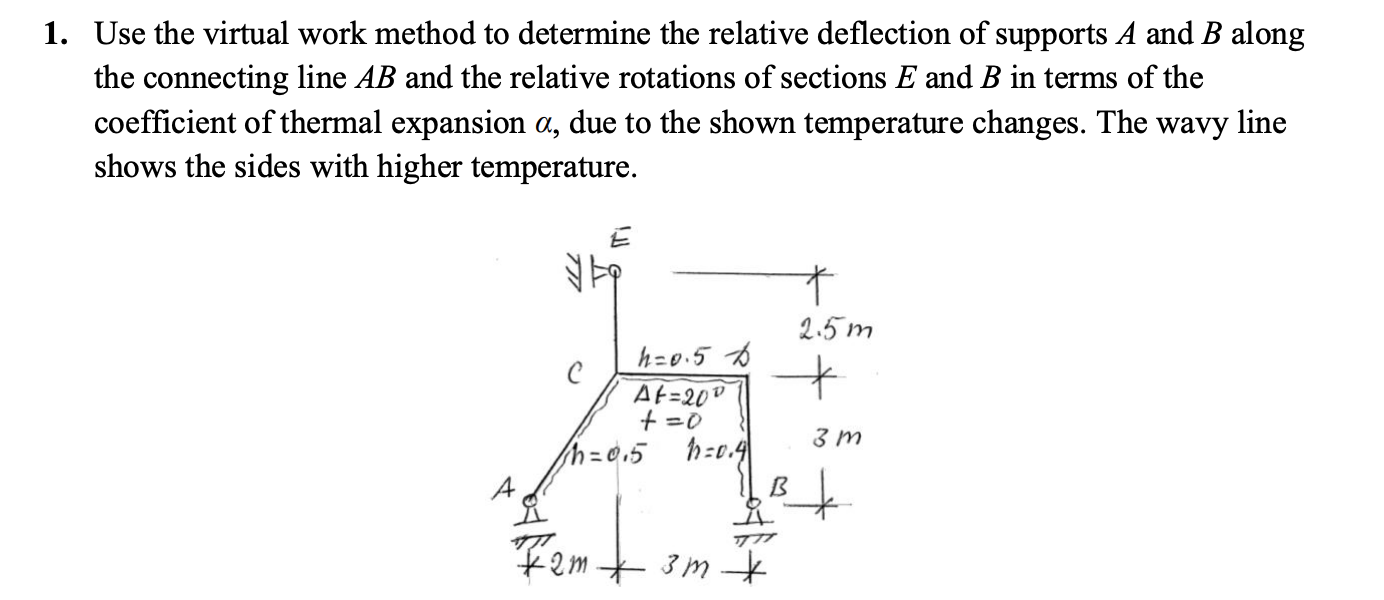# Question 1. Use the virtual work method to determine the relative deflection of supports A and B along the connecting line AB and the relative rotations of sections E and B in terms of the coefficient of thermal expansion a, due to the shown temperature changes. The wavy line shows the sides with higher temperature. ta t 2.5m t h=0.5 D C At=20V +=0 h=0.5 10.41 3 m By kom 3 mRVBPPV The Asker · Civil EngineeringTranscribed Image Text: 1. Use the virtual work method to determine the relative deflection of supports A and B along the connecting line AB and the relative rotations of sections E and B in terms of the coefficient of thermal expansion a, due to the shown temperature changes. The wavy line shows the sides with higher temperature. ta t 2.5m t h=0.5 D C At=20V +=0 h=0.5 10.41 3 m By kom 3 m
More
Transcribed Image Text: 1. Use the virtual work method to determine the relative deflection of supports A and B along the connecting line AB and the relative rotations of sections E and B in terms of the coefficient of thermal expansion a, due to the shown temperature changes. The wavy line shows the sides with higher temperature. ta t 2.5m t h=0.5 D C At=20V +=0 h=0.5 10.41 3 m By kom 3 m